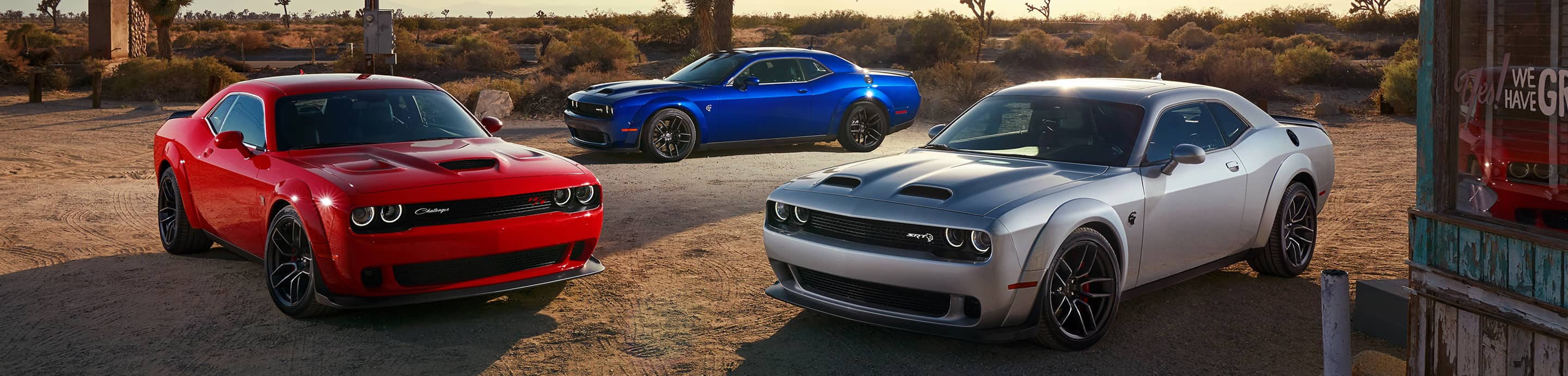1 - 12 of 12 Posts

#### mauls

·
##### Banned
Joined
·
675 Posts
Discussion Starter · ·
They call the 6.4L a 392ci, but I see people running 370 decals for their 6.1L when it should be 372?

Just curious

#### hemi 05

·
##### Registered
Joined
·
198 Posts
Im not sure but I think the 6.1 is actually closer to 370 than 372 but someone that knows for sure will probably chime in.

#### a14umbra

·
Joined
·
1,042 Posts
I'm not sure, but this may be the reason. You may be looking at it from the wrong direction. Perhaps it is truly 370C.I.D.

370 cubic inches = 6.06321368 liters

You're not going to call it a 6.06321368L engine, so it's rounded up to 6.1L.

GEEK TIME

The 6.1 bore and stroke are:

BORE = 4.055 in
STROKE = 3.58 in

To calculate the displacement of one cylinder its Height*Pi*radius squared.
The diameter (bore) is 4.055, so the radius is half of that or 2.0275.
To square that we multiply 2.0275*2.0275 = 4.11075625.
That takes care of radius squared. Multplying by pi is 4.11075625*3.14159265953 = 12.9143216356991774375.
Now muliply that by the height (stroke). 12.9143216356991774375*3.58 = 46.23327145580305522625 cubic inched per cylinder.
So mulitply that by 8 cylinders and you get 369.86617164642444181 cubic inches which is 6.06102063 liters.

369.86617164642444181 cubic inches rounds to 370 C.I.
6.06102063 liters rounds to 6.1L

Mystery solved (I think)

#### mauls

·
##### Banned
Joined
·
675 Posts
Discussion Starter · ·
^^ Good enough for mewas just wondering

Thanks guys

#### gr8srt8

·
##### Registered
Joined
·
1,658 Posts
^^ Good enough for mewas just wondering

Thanks guys
Now the resulting question:

Why is the subfolder named: 6.1L / 372ci(2008 - 2009)?

"I know that is where you're going..."

#### a14umbra

·
Joined
·
1,042 Posts
Now the resulting question:

Why is the subfolder named: 6.1L / 372ci(2008 - 2009)?

"I know that is where you're going..."
Doh! Never noticed that.

·
Joined
·
3,881 Posts
4.055 x 3.579

#### a14umbra

·
Joined
·
1,042 Posts
4.055 x 3.579
DAMMIT! Now I have to start all over again.

BORE = 4.055 in
STROKE = 3.579 in

To calculate the displacement of one cylinder its Height*Pi*radius squared.
The diameter (bore) is 4.055, so the radius is half of that or 2.0275.
To square that we multiply 2.0275*2.0275 = 4.11075625.
That takes care of radius squared. Multplying by pi is 4.11075625*3.14159265953 = 12.9143216356991774375.
Now muliply that by the height (stroke). 12.9143216356991774375*3.579 = 46.2203571341673560488125 cubic inched per cylinder.
So mulitply that by 8 cylinders and you get 369.7628570733388483905 cubic inches which is 6.0593276 liters.

369.7628570733388483905 cubic inches rounds to 370 C.I.
6.0593276 liters rounds to 6.1L

#### mauls

·
##### Banned
Joined
·
675 Posts
Discussion Starter · ·
Now the resulting question:

Why is the subfolder named: 6.1L / 372ci(2008 - 2009)?

"I know that is where you're going..."
yup that was my next question haha

#### skialfs

·
##### Registered
Joined
·
2,311 Posts
Now the resulting question:

Why is the subfolder named: 6.1L / 372ci(2008 - 2009)?

"I know that is where you're going..."
Because it is wrong...

#### homerzeppelin

·
##### Registered
Joined
·
301 Posts
I always through the 370 was for 370HP for the 5.7, haha.

1 - 12 of 12 Posts